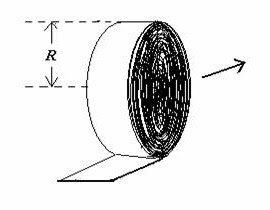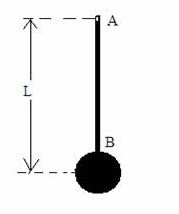## Pages

`“Life is like riding a bicycle.  To keep your balance you must keep moving.”–Albert Einstein`

## Saturday, July 16, 2011

### AP Physics C – Circular Motion and Rotation - Multiple Choice Practice Questions

“Our greatest weakness lies in giving up. The most certain way to succeed is always to try just one more time”.
– Thomas A. Edison
Questions on circular motion and rotation were discussed earlier on this site. You can access them by clicking on the label ‘circular motion and rotation’ below this post’. Today we will discuss a few more interesting multiple choice practice questions in this section:(1) A long, flexible ribbon of mass M rolled in the form of a cylinder (Fig.) of radius R is placed on horizontal floor. When a negligibly small push is applied, the ribbon unrolls without sliding along the surface (thereby reducing the radius of the cylinder). The horizontal velocity of the axis of the cylinder when the radius becomes (¾)R is
(a) √(2Rg)
(b) √(3Rg/2)
(c) √(27Rg/17)
(d) √(37Rg/27)
(e) √(47Rg/37)
When the roll of ribbon unrolls, the radius of the cylindrical roll decreases, thereby lowering the centre of gravity of the cylinder. Initially the mass of the cylinder is M and its centre of gravity is at a height R. When the radius of the cylinder becomes (¾)R, the mass of the cylinder is (9/16) M and its centre of gravity is at a height (¾)R
[When the radius is R, mass = M = πR2 where L is the length and ρ is the density of the cylinder. When the radius is (¾)R, mass = π(9/16)R2= (9/16) M].
The initial gravitational potential energy of the cylinder is MgR and the final gravitational potential energy is (9/16)Mg×(¾)R = (27/64)MgR.
Therefore the loss of potential energy = MgR (27/64)MgR = (37/64)MgR
The cylinder gains an equal amount of kinetic energy (partially rotational and partially translational) which is ½ Iω2 + ½ mv2 where I is the moment of inertia [of the cylinder of radius (¾)R] about its axis, ω is its angular velocity, m is its mass and v is its linear velocity. Thus we have
(37/64)MgR = (½)(mr2/2)(v2/r2 )+ ½ mv2 = (¾)mv2
[Remember that the moment of inertia of a cylinder of mas m and radius r about its own axis is mr2/2 and the angular velocity is v/r where v is the linear velocity].
Therefore, (37/64)MgR = (¾)(9/16)Mv2
This gives v = (37Rg/27), as given in option (d).
[The above question is perhaps more suitable as part of a free response question].
(2) The angular momentum of a fly-wheel changes from 2L to 5L in 5 seconds under the action of a constant torque. The torque is
(a) 5L
(b) 3L
(c) 2L
(d) (3/5)L
(e) (2/5)L
The change in the angular momentum of the fly-wheel is 3L. The angular impulse received by the fly-wheel during the time t is τ t where τ is the torque. Since the angular impulse is equal to the change in angular momentum, we have
τ t = 3L
Since t = 5 seconds, τ = (3/5)L(3) The adjoining figure shows a bar pendulum. It consists of a sphere of mass M attached to the end B of a light rigid rod AB suspended from a horizontal nail (at A) on the wall. Initially the pendulum is at rest in its equilibrium position, with the rod vertical. What is the magnitude of the minimum velocity to be imparted to the sphere so that it just completes its circular path?
(a) √(gL)
(b) √(2gL)
(c) √3gL)
(d) √(4gL)
(e) √(5gL)
Since the rod is light, this problem is quite simple. It is enough to equate the initial kinetic energy of the sphere to its potential energy (gravitational) at its topmost position. Therefore we have
½ Mv2 = Mg×2L where ‘v’ is the magnitude of the minimum velocity to be imparted to the sphere.
[Note that the height through which the centre of gravity of the sphere rises is 2L].
Therefore, v = √(4gL)
[If the sphere is suspended by means of a string (as in the case of a simple pendulum), the minimum speed to be imparted will be greater {equal to √(5gL)}, since the sphere should possess kinetic energy also at its topmost position, in order to maintain its circular path. In that case the tension in the string is zero and the speed at the top of the path (vtop) is such that the centrifugal force on the sphere has the same magnitude as the weight of the sphere:
Mvtop2/L = Mg from which vtop = √(gL)
The kinetic energy at the top is ½ Mvtop2 = ½ MgL
The minimum kinetic energy to be imparted to the sphere in its initial position is ½ MgL + (Mg×2L) = (5/2) MgL
Therfore, the minimum velocity ‘v’ to be imparted to the sphere is given by
½ Mv2 = (5/2) MgL from which v = √(5gL)](4) A simple pendulum of length L has a bob of mass M. The pendulum is suspended from the point O and is held initially in the position OA, as shown in the adjoining figure, so that the string is horizontal. On releasing the pendulum, what will be the resultant acceleration of the bob when the pendulum is in the position OB? (Acceleration due to gravity = g)
(a) g [1 + 3 sin2θ]1/2
(b) g [1 + 3 cos2θ]1/2
(c) 3g sin2θ
(d) 3g (1+sinθ)
(e) 3g/2
The forces acting on the bob of the pendulum in the position OB are the weight Mg of the bob acting vertically downwards and the tension T (Fig.) in the string which supplies the centripetal force required for the circular motion of the bob.The speed v of the bob in the position B is given by
½ Mv2 = Mg Lsinθ
Therefore v = (2gL sinθ) ……….(i)
[In position B the bob has fallen down by a distance L sinθ, losing gravitational potential energy mg Lsinθ and gaining kinetic energy ½ Mv2]
The centripetal force on the bob is the resultant of the tension T in the string and the component Mg sinθ of the weight of the bob. Therefore we have
Mv2/L = T Mg sinθ
Substituting for v from Eq.(i) we have
2Mg sinθ = T Mg sinθ
This gives T = 3Mg sinθ
The resultant force F acting on the bob is the resultant of the forces Mg and T (with an angle 90º+θ between them) and has magnitude equal to [(Mg)2 + T2 + (2×Mg× T) cos(90+θ)]1/2.
Substituting for T, the resultant force is
F = [(Mg)2 + (3Mg sinθ)2 + (2× Mg× 3Mg sinθ) cos(90+ θ)]1/2 = [(Mg)2 + 3(Mg sinθ)2]1/2 = Mg [1 + 3 sin2θ]1/2
[Remember that cos(90+ θ) = sinθ]
The resultant acceleration of the bob in position B is F/M = g [1 + 3 sin2θ]1/2.

(5) A disc of mass M and radius R and a ring of mass M and radius 2R are released from rest from the top of an inclined plane. If they roll down the plane without slipping, which one of the following statements is correct?
(a) The ring will reach the bottom of the incline earlier
(b) They will reach the bottom of the incline with the same speed
(c) The ring will reach the bottom of the incline with twice the speed of the disc
(d) They will have the same acceleration down the incline
(e) They will reach the bottom of the incline with the same kinetic energy
The acceleration ‘a of a body of radius R rolling down an incline of angle θ is given by
a = g sinθ/[1 + k2/R2] where k is the radius of gyration of the body.
[The radius of gyration k is defined by I = Mk2 where I is the moment of inertia (about the axis of rolling) and M is the mass].
Note that the acceleration is independent of the mass and size of the body. The ratio k2/R2 also is independent of the mass and size of the body
For a given inclined plane, the acceleration depends only on the ratio k2/R2.

For a given inclined plane, the acceleration depends only on the ratio k2/R2.

[You may substitute the value of moment of inertia in the equation I = Mk2 and find the value of k2/R2. The maximum value in the case of regularly shaped bodies is for a thin ring and is equal to 1 since I = MR2 = Mk2. The least value is 2/5 which is the case for a solid sphere since I = (2/5)MR2. Better, see this post ]
Moment of inertia of a disc (about the axis of rolling) is MR2/2 where as the moment pf inertia of a ring (about the axis of rolling) is MR2.

The acceleration in the case of a disc is g sinθ/[1+ ½] = (2/3) g sinθ

The acceleration in the case of a ring is g sinθ/[1+ 1] = (½) g sinθ

All options except the last one (e) in the question are wrong. The kinetic energy with which the disc and the ring will reach the bottom of the incline will be the same since they have the same mass and they start from the same height.

[The kinetic energy at the bottom of the incline is the same as the potential energy at the top of the incline].